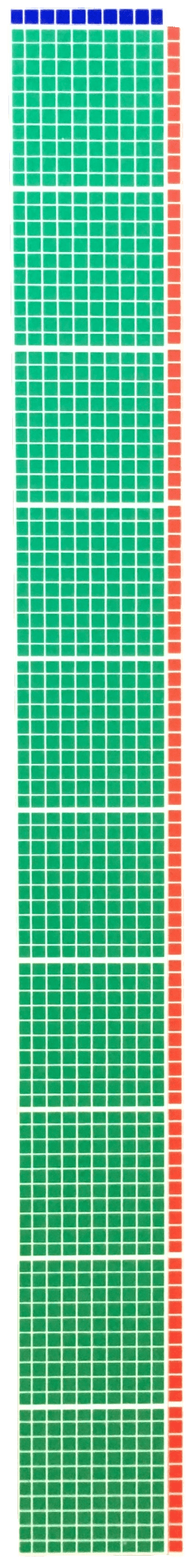# Quotient Rule – Same Base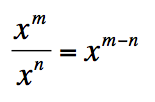###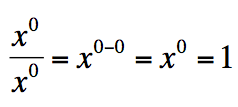1 divided by 1 = 1###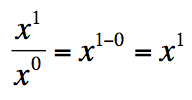x divided by 1 = x###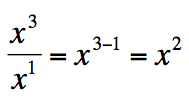x cube divided by x is x square